JEE  >  MCQs (One or More Correct Options): Rotational Motion

# MCQs (One or More Correct Options): Rotational Motion - Notes | Study All Types of Questions for JEE - JEE

 1 Crore+ students have signed up on EduRev. Have you?

Q.1. A solid sphere is resting over a rough horizontal floor. A sharp impulse is applied on it along its horizontal diameter. Point out false statements:
(a) The kinetic energy of the sphere remains constant throughout the motion
(b) The kinetic energy of translation is shared by rotation, but the total kinetic energy decreases initially and finally attains a constant value
(c) The total kinetic energy of the sphere decreases continuously on doing work against friction and finally reduces to zero
(d) A constant frictional force, opposite to the translational motion acts on the sphere throughout its motion.

Correct Answer is option (a, c and d)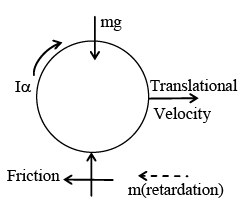Since, the impulse is applied along a horizontal diameter, therefore, due to that impulse the sphere starts to move translationally without any rotational motion. Since the floor is rough, therefore, friction comes into existence and that opposes forward sliding of the sphere as shown in figure. Hence, friction acts along backward direction which not only provides a retarding force but produces an accelerating moment also. Due to that moment the sphere experiences an angular acceleration. Hence, initially translational velocity of the sphere decreases but angular velocity increases. Therefore, KE of the sphere initially decreases. Rotational motion is accelerated till angular velocity (ω) becomes equal to r v and then the friction disappears. Hence, then total energy of sphere remains constant. Therefore, option (B) alone is correct and rest options are incorrect.

Q.2. A ring of mass M and radius R sliding with a velocity v0 suddenly enters into rough surface where coefficient of friction is μ, as shown -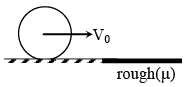(a) The ring starts rolling motion when centre of mass becomes stationary
(b) The ring starts rolling motion when the point of contact becomes stationary.
(c) The time after which the ring starts rolling is v0/2μg
(d) The rolling velocity is v0/2

Correct Answer is option (b, c and d)
Let rolling velocity is v and angular velocity is ω then,
v = v0 – μgt  .... (1)
and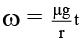.... (2)

Also, v = rω
∴ μgt = v0 - μgt ⇒ t = v0/2μg and v = v0/2

Q.3. A cylinder of mass m is kept on a inclined plane having angle of inclination 30º. Axis of cylinder makes an angle 30º with line along greatest slope. Assuming that cylinder rolls without slipping -
(a) Acceleration of cylinder is g/6
(b) Acceleration of cylinder is g/4
(c) Friction force acting on cylinder is √7mg/3

(d) Friction force acting on cylinder is √7mg/6

Correct Answer is option (a and d)
Acceleration of cylinder is given by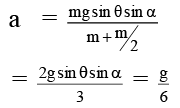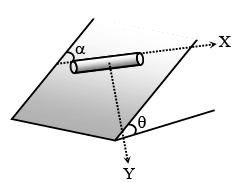Friction along axis: fx = mg sin θ cos α
Friction ⊥ to axis: fy =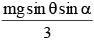∴ Friction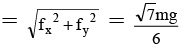Q.4. A uniform rod of mass 1 kg and length 1 m is kept vertical on the edge of a horizontal table. The rod is hit at a height 75 cm from table surface as to provide impulse 5 N/S in horizontal direction. Then -
(a) Displacement of rod after 1 sec is 5√2 m
(b) Initial velocity of rod is 5 m/s which increases with time
(c) Initial angular velocity of rod is 15 rad/sec which increases with time
(d) Numbers of rotation in 1 sec is 15/2π

Correct Answer is option (a, b and d)
Just after impulse -
Velocity of centre of mass = 5 N/s/1kg = 5m/s (Horizontal)
Angular velocity of rod =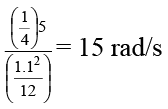∴ Displacement of centre of mass =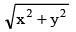[x = Displacement in horizontal direction, y = Displacement in vertical direction]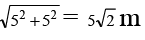Angular velocity of rod will not increase with time as there is no angular acceleration about axis passing through centre of mass (Torque of mg about centre of mass is zero as it acts through centre of mass)

Q.5. Work done by a force on an rigid object having no rotational motion will be zero, if -
(a) the force is always perpendicular to acceleration of object.
(b) the object is at rest relative to ground but the point of application of force moves on the object.
(c) the force is always perpendicular to velocity of object.
(d) the point of application of force is fixed relative to ground by the object moves.

Correct Answer is option (b and c)
(a) If velocity and acceleration are not in same direction. Work done by force perpendicular to acceleration will not be zero.
(b) If the object is at rest no force can do work.
(c) If force is perpendicular to velocity work done will be zero.
(d) If the point on the body has velocity component in direction of application of force work done will be non-zero.

Q.6. A wheel rolls purely between a rough horizontal surface below it and a horizontal plank above it under the action of a horizontal force F applied on the plank. If at any time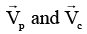represents velocity of plank and velocity of centre of mass of wheel and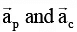represents acceleration of plank and acceleration of C.M. of wheel respectively then which of the following is/are correct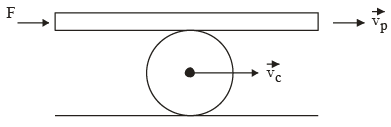(a)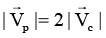(b)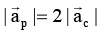(c)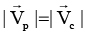(d)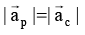Correct Answer is option (a and b)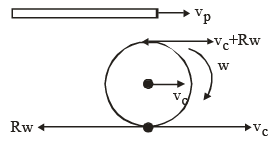For pure rolling of wheel w.r.t. horizontal surface below it vc = Rw For pure rolling of wheel w.r.t. plank

vp = vc + Rw = 2vc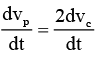aP =  2ac

Q.7. Four identical rods, each of mass m and length l, are joined to form a rigid square frame. The frame lies in the xy plane, with its centre at the origin and the sides parallel to the x and y axes. Its moment of inertia about.
(a) the x-axis is 2/3 ml2

(b) the z-axis is 4/3 ml2
(c) an axis parallel to the z-axis and passing through a corner is 10/3 ml2

(d) one side is 5/2 ml2

Correct Answer is option (a, b, c and d)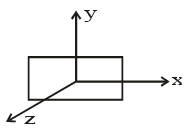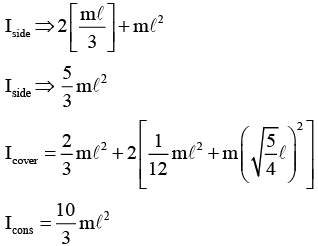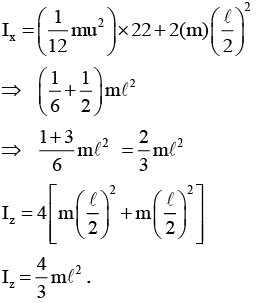Q.8. A disc is given an initial angular velocity ω0 and placed on rough horizontal surface as shown. The quantities which will not depend on the coefficient of friction is/are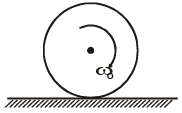(a) The time until rolling begins.
(b) The displacement of the disc until rolling begins.
(c) The velocity when rolling begins.
(d) The work done by the force of friction.

Correct Answer is option (c and d)
The velocity of the disc when rolling begins can be obtained using the conservation of angular momentum principle about the point through which the friction force acts. So, the coefficient of friction has no bearing on final velocity. The work done by the force of friction will simply be change in kinetic energy.

Q.9. A thin rod of mass ’m’ and length ‘l’ rotates freely about one of its ends. The other end held upwards and released when it makes an angle 'θ' with the vertical and top edge acquires a maximum velocity of ums-1. Then
(a) The maximum change in PE is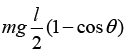(b) The angle (θ) from which it released is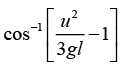(c) The angle (θ) from which it released is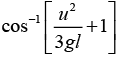(d) The maximum change in PE is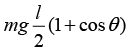Correct Answer is option (b and d)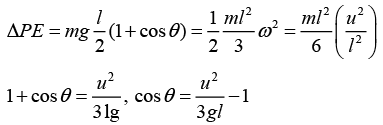Q.10. A particle and a pivoted rod, lie on a horizontal smooth plane. The particle moves perpendicular to length of rod as shown in the diagram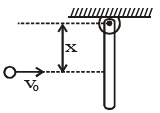If the length of rod is L and normal contact force developed at hinge is N then
(a) For x = 2L/3, N ≠ 0 & forward on rod
(b) For x = L/3, N ≠ 0 & backward on rod
(c) For x = L, N ≠ 0, Forward on rod
(d) Total linear momentum of rod – ball system can increase or decrease depending upon the value of ‘x’.

Correct Answer is option (a, b and d)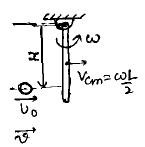For no Normal contact fore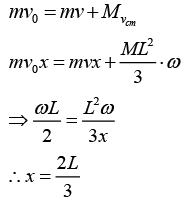The document MCQs (One or More Correct Options): Rotational Motion - Notes | Study All Types of Questions for JEE - JEE is a part of the JEE Course All Types of Questions for JEE.
All you need of JEE at this link: JEE

376 docs

## All Types of Questions for JEE

376 docs

Track your progress, build streaks, highlight & save important lessons and more!

,

,

,

,

,

,

,

,

,

,

,

,

,

,

,

,

,

,

,

,

,

;